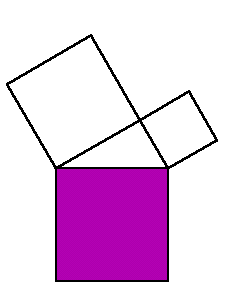This animated illustration of the Pythagorean Theorem was inspired by a comment in the problem section of Chapter 5 of "An Introduction to the History of Mathematics" by Howard Eves, Sixth edition, Saunders, 1990.  The square on the hypotenuse is divided into two rectangles by dropping a perpendicular from the vertex with the right angle.  The transformation of each rectangle into a parallelogram and finally into a square on one leg preserves area.